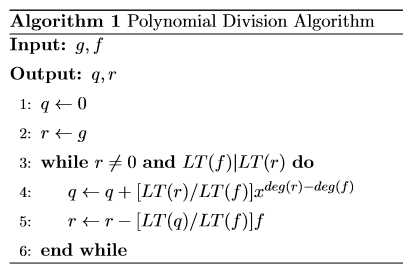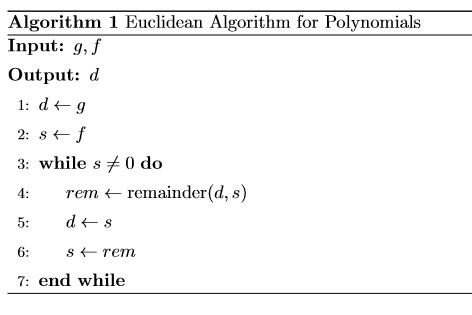## 交换环

• 对所有$a,b \in R$，有$a+b=b+a$
• 对所有$a,b,c \in R$，有$a+(b+c)=(a+b)+c$
• 存在元素$0 \in R$，使得对所有$a \in R$，有$0+a=a$
• 对每一个$a \in R$，都有$a’ \in R$使得$a’+a=0$(即$(R,+)$是一个阿贝尔群)
• 对所有$a,b \in R$，有$a \cdot b=b \cdot a$
• 对所有$a,b,c \in R$，有$a \cdot (b \cdot c)=(a \cdot b) \cdot c$
• 存在元素$1 \in R$，使得对每一个$a \in R$，都有$1 \cdot a=a$
• 对所有$a,b,c \in R$，都有$a \cdot (b+c) = a \cdot b+a \cdot c$

### 性质

• 对每一个$a \in R$，有$0 \cdot a=0$
• 若$-a$满足$-a+a=0$，那么$(-1) \cdot (-a) = a$
• 对每一个$a \in R$，有$(-1) \cdot a =-a$
• 对所有$a,b \in R$，定义$a-b = a+(-b)$

## 环

• $(R,+)$是一个阿贝尔群，且运算$+$的单位元是$0$
• 对所有$a,b,c \in R$，都有$a \cdot (b+c) = a \cdot b+a \cdot c,(b+c) \cdot a=b \cdot a+c \cdot a$
• $(R, \cdot )$满足结合律(但不一定可交换)

## 零环与整环

• $1 \neq 0$
• 若$c \cdot a=c \cdot b$，且$c \neq 0$，则$a=b$(消去律)

## 子环

• $1 \in S$
• 若$a,b \in S$，那么$a-b \in S$
• 若$a,b \in S$，那么$ab \in S$

## 函数上的交换环

$\mathcal{F} (\mathbb{R})$中的0元为$z(a)=0, a \in \mathbb{R}$
$\mathcal{F} (\mathbb{R})$中的1元为$\epsilon (a)=1, a \in \mathbb{R}$

## 同余类上的交换环

• $\mathbb{I}_{m}$是一个交换环
• $\mathbb{I}_{m}$是整环当且仅当$m$是质数

## 交换环除法

### 性质

• 若$a \mid b$且$b \mid c$，那么$a \mid c$
• 若$a \mid b$且$a \mid c$，那么$a$可以整除每一个可以用$sb+tc$表示的元素，其中$s,t \in R$

## 可逆元

### 性质

• 可逆元的逆唯一
• 若u是可逆元，则对任何$w \neq 0$，有$uw \neq 0$
• R中的可逆元组成的集合对运算$\cdot$形成阿贝尔群

### 定理

• 设$R$是一个整环，若元素$a,b \in R$非零，那么$a \mid b$且$b \mid a$当且仅当对某个可逆元$u \in R$，有$b=ua$
• 若a是整数，则$[a]$是$\mathbb{I}_{m}$的可逆元当且仅当$gcd(a,m)=1$
• 若$p$是质数，那么$\mathbb{I}_{p}$每一个非零的$[a]$都是可逆元

## 域

### 定理

• 每一个域$F$都是一个整环
• 交换环$\mathbb{I}_{m}$是一个域当且仅当$m$是质数
注:当$p$为质数时，$\mathbb{I}_{p}$也可表示为$\mathbb{F}_{p}$

### 分式域

• 对所有$a,b \in R, b \neq 0$，定义$F={[a,b]}$
• 定义相等:若$ad=bc$，则$[a,b]=[c,d]$
• 定义$+$运算为$[a,b]+[c,d]=[ad+bc,bd]$
• 定义$\cdot$运算为$[a,b] \cdot [c,d]=[ac,bd]$
• 定义$[0,1]$，或$[0,b],b \neq 0$为零元
• 定义$[1,1]$，或$[b,b],b \neq 0$为一元

$F$是域请自行证明

$Frac(\mathbb{Z})=\mathbb{Q}$

## 子域

### 定理

• 域$K$的子集$k$是一个子域，当且仅当$k$是一个对逆元运算封闭的子环，即若$a \in k$并且$a \neq 0$，那么$a^{-1} \in k$
• 若$\lbrace F_{i}:i \in I \rbrace$是域$K$的任何子域所构成的集合簇，那么$k=\bigcap_{i \in I} F_{i}$也是$K$的一个子域
注:若$K$是一个域，那么$K$的所有子域的交集$k$被称作$K$的素域

## 多项式

$s_{i} \in R$被称为序列的系数，序列$\sigma = \tau$当且仅当$\sigma (i)= \tau (i),i \geq 0$

## 多项式环

### 定理

• 若$R$是交换环，那么$R[x]$也是交换环且$R$是其子环
• 若$R$是整环，那么$R[x]$也是整环

• Either $\sigma \tau =0$ or $deg(\sigma \tau) \leq deg(\sigma) + deg(\tau)$
• 若$R$是一个整环，那么$\sigma \tau \neq 0$且$deg(\sigma \tau) = deg(\sigma) +deg(\tau)$

## 未知数

### 定理

• 若$\sigma=(s_{0},s_{1}, \cdots,s_{j},\cdots)$，那么$x \sigma=(0,s_{0},s_{1}, \cdots, s_{j}, \cdots)$
• 若$n \geq 1$，则$x^{n}$的各个元素全是0，除了第n个元素为1
• 若$r \in R$，则$(r,0,0, \cdots)(s_{0},s_{1}, \cdots ,s_{j}, \cdots)=(rs_{0},rs_{1}, \cdots ,rs_{j}, \cdots)$

## 多项式函数

### 定理

• $F(x)$中的元素有$f(x)/g(x)$的形式，其中$f(x),g(x) \in F[x]$且$g(x) \neq 0$
• 若$p$是素数，那么有理函数域$\mathbb{F}_{p} (x)$是一个有限域，其素域为$\mathbb{F}_{p}$

## 多变量多项式

$R[x_{1},x_{2}, \cdots, x_{n}]$也类似

## 环同态

• $f(1)=1$
• 对所有$a,a’ \in A$有$f(a+a’)=f(a)+f(a’)$
• 对所有$a,a’ \in A$有$f(aa’)=f(a)f(a’)$

### 性质

• 对所有$n \geq 0$，有$f(a^{n})=f(a)^{n}$
• 若$a$是一个可逆元，那么$f(a)$也是一个可逆元并且$f(a^{-1})=f(a)^{-1}$
• 若$a$是一个可逆元，那么对所有$n \geq 1$有$f(a^{-n})=f(a)^{-n}$
• 若定义$U(A)$为$A$中的可逆元组成的群，有$f(U(A)) \subseteq U(R)$，且若$f$是一个同构，有$U(A) \cong U(R)$

### 核与像

• $0 \in \mathbf{ker}f$
• 若$x,y \in \mathbf{ker} f$，有$x+y \in \mathbf{ker} f$
• 若$x \in \mathbf{ker} f$且$a \in A$，有$ax \in \mathbf{ker}f$

## 理想

• $0 \in I$
• 若$a,b \in I$，那么$a+b \in I$
• 若$a \in I$且$r \in R$，那么$ra \in I$

### 主理想

$\mathbb{Z}$上的每一个理想都是主理想

### 推论

• 环同态$f: A \to R$是单射当且仅当$\mathbf{ker} f =\lbrace 0 \rbrace$
• 若$f:k \to R$是环同态，且$R$不是一个零环，且当$k$是一个域，那么$f$是一个单射

### 理想与环

• 若$R$是交换环，且存在可逆元$u \in R$使得$a=ub$，那么$(a)=(b)$
• 若$R$是一个整环，那么$(a)=(b)$可以推出存在可逆元$u \in R$使得$a=ub$
• 非零交换环$R$是一个域，当且仅当它仅有的理想是$\lbrace 0 \rbrace$和$R$
• 交换环$R$上的理想$\lbrace 0 \rbrace$是一个素理想，当且仅当$R$是整环
• $(p)$为$\mathbb{Z}$上的素理想，且有$p=0$或$p$是一个素数

## 多项式除法算法

• 有多项式$q(x),r(x) \in R[x]$使得$g(x)=q(x)f(x)+r(x)$，其中有$r(x)=0$或$deg(r) < deg(f)$
• 若$R$是一个整环，那么上文中提到的$q(x)$和$r(x)$是独特的## 多项式的根

### 定理

• 若$f(x)$的次数为$n$，那么$f(x)$在$k$上最多有$n$个根
• 若$f(x)$的次数为$n$，并且$a_{1},a_{2}, \cdots ,a_{n} \in k$是$f(x)$的独特的根，那么有$c \in k$满足$f(x)=c(x-a_{1})(x-a_{2}) \cdots (x-a_{n})$

## 多项式的最大公约式

### 定理

• 一个首一的公约式$d(x)$是最大公约式，当且仅当$d(x)$能整除每一个公约式
• 任何两个多项式$f(x)$和$g(x)$都有唯一的最大公约式

### 欧几里得算法## 欧氏环

• 对所有$f,g \in R^{\times}$，有$\vartheta (f) \leq \vartheta (fg)$
• 对所有$f,g \in R$，其中$f \in R^{\times}$，那么存在$q,r \in R$满足$g=qf+r$，且有$r=0$或$\vartheta (r) < \vartheta (f)$

$R^{\times}$表示$R$中所有非零元素组成的集合

### 举例

• 每一个域$R$都是欧氏环，且$\vartheta =0$
• $\mathbb{Z}$是欧氏环，且对$m \in \mathbb{Z}$，有$\vartheta (m)= \mid m \mid$
• 对一个域$k$，$k[x]$是欧氏环，且对$f \in k[x]$，有$\vartheta(f)=deg(f)$

## 商环

$R/I$上的加法和乘法运算定义如下:

$\mathbb{I}_{m}$上的同余类也可以用如下形式表示:

## 极大理想

### 举例

• $\lbrace 0 \rbrace$是$R$上的极大理想，当且仅当$R$是域
• 如果$R$是一个主理想整环，那么每一个非零素理想都是极大理想